# Discrete Optimization: beyond REINFORCE

### Gumbel Softmax (a.k.a. Concrete distribution)

While it might still be too early to start talking about the next A.I. winter, researchers are starting to see the limits of machine learning’s current set of tools as they start to exhaust the publication fruits of backpropagation. Intuitively, it feels like we as human often think in discrete units of thought and make discrete decisions. However, the current paradigm of fully differentiable supervised learning (A.I.’s main name to fame in the current age) doesn’t allow us to make discrete choices in our model.

For the case in point, imagine your model outputs a multinoulli distribution of actions with probabilities:

Note in practice the π’s would be represented using a vector. In supervised learning, you’re given a label π* (represented as a 1-hot vector) and the model learns by making the predictions π_1, π_2, … as close to π* as possible for example using the cross-entropy loss. However, it’s not always the case that we have labels for our actions. In real life, we make choices without someone telling us exactly what we should and are rewarded at later time based on our choices. In other words your environment requires you to pick a discrete action so that you can act the in world and be rewarded. But making a discrete choice is not differentiable, how can we learn using backpropagation?

Making a discrete choice then being rewarded later comes up in a lot of places in machine learning. For example when I worked on image captioning, my model had to make a discrete choice on the next word in the sentence at each time step. Only when the entire sentence was constructed could I evaluate it and send a learning signal back to the network. Making discrete choices also has the potential to unlock a whole domain of algorithms and ideas people haven’t tried before. Consider image classification. One intuitive way to improve classification is by invoking “support images.” Just as a human would go to Google to look at similar images when asked to classify a breed of dog, a model that can utilize support images should outperform one which does not. Choosing a support image from a set of possible images is a discrete choice and therefore non-differentiable.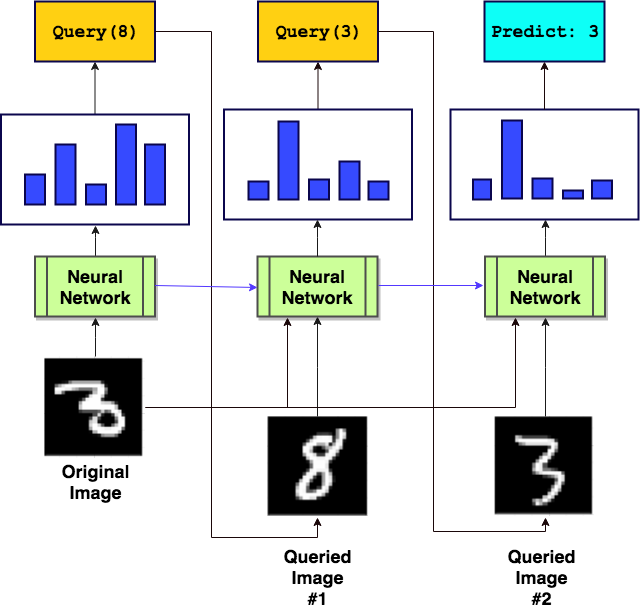An example of a neural network that makes queries for “support images” to better classify images. For classes that look similar (8 and 3) it will benefit the network to query for examples belonging to each class to compare against the original image. Courtesy of @SirrahChan

Typically problems with discrete choices are solved using the REINFORCE algorithm. REINFORCE works by taking a sample of our π distribution (represented as a 1-hot vector). The environment tells us whether our sample did well or not and we learn by encouraging the good actions and discouraging the bad actions. Put colloquially, if supervised learning is baking a batch of cookies and being told exactly how the recipe needs to change to improve the cookies, REINFORCE is baking a batch of cookies and being told whether the cookies were good or not: how the recipe needs to change is not specified. Just from our analogy, we can see how learning with REINFORCE can be problematic. REINFORCE suffers from high variance and has been shown to have bad empirical performance in certain situations.

Gumbel softmax or Concrete provides an alternative to REINFORCE where we make a differentiable approximation to a discrete sample by sampling a 0.9999-hot vector instead of a 1-hot vector. When the environment is known and differentiable, Gumbel softmax allows us to backpropagate directly from the reward to the parameters of the model instead of using REINFORCE. When the environment is not differentiable, it’s not clear whether Gumbel softmax will do better than REINFORCE but there is empirical evidence to suggest so. For those familiar with the Reparameterization trick, Gumbel softmax looks a lot like it but for discrete latent variables.

Recall that REINFORCE samples a 1-hot vector from our π distribution. To construct our 0.9999-hot vector for Gumbel softmax, we need to select values for each index in the vector. The ith element in our 0.9999-hot action vector y is determined by the following equation:

As you’ll notice, this is the softmax function applied to this weird log(π_i + g_i) term. τ is the temperature of the softmax. The g_i term is actually a random value drawn from the Gumbel distribution which is just −log(−log(u_i)) where u_i is drawn from a uniform distribution. Hence the name Gumbel softmax. There’s also a “Gumbel hardmax” equation which takes the argmax of the indices instead of applying a softmax function:

It turns out that by taking a “Gumbel hardmax” sample, we are directly sampling from the multinoulli distribution π (this is perhaps not super obvious because we’ve introduce logs and g_i in the sampling process). Therefore, the soft version approximately samples from the multinoulli distribution. Sampling from the Gumbel softmax is an approximation to sampling from the multinoulli distribution for 2 reasons. First, the Gumbel softmax makes a sample with k nonzero elements instead of 1 nonzero element. Remember k is the number of possible actions (dimension of action space). Therefore a sample from Gumbel softmax is never exactly a sample from the multinoulli. Second, the expected value of Gumbel softmax samples is not equal to the expected value of the multinoulli samples unless the temperature τ goes to 0 (at which point Gumbel softmax becomes “Gumbel hardmax”). In the face of all these approximations you might be slightly skeptical as to why the Gumbel softmax woud work at all but it has been shown to have good empirical results. For a more theoretical argument, let’s revisit the age-old bias-variance trade off. Here is what a sample from the Gumbel softmax distribution looks like for different temperatures: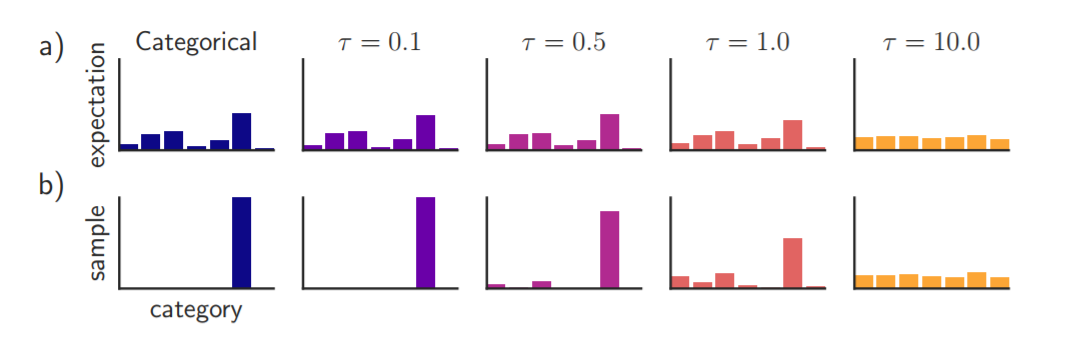Visualization of a typical sample for different gumbel temperatures and the expected distribution of the samples for that temperature.

In our visualization, the bottom row shows a typical sample from the Gumbel softmax distribution. As we can see, a typical sample at low temperatures is almost 1-hot. The top row shows the expected value of the sample. At low temperatures, this is very close to the actual expected value of the multinoulli/categorical distribution. At high temperatures, entropy dominates and the expected value is almost uniform and no longer approximates the multinoulli very well. Moreover, the samples themselves are almost uniform. This is just the age old bias-variance trade off stated another way. As we increase the temperature, our samples become more and more biased (expected value deviates from true expected value) but the variance of our samples decrease (since samples approach the uniform distribution). This is analogous to using L2 regularization for neural networks. As we increase the regularization, we increase the bias but reduce the variance of our model weights. And from what we know about L2 regularization, there’s usually a sweet spot where the trade off between bias and variance gives us optimal performance. This is also partly true for Gumbel softmax: use too low of a temperature and you’re effectively doing REINFORCE, use too high of a temperature and you won’t learn because your samples don’t represent your π distribution. To hit home the point, if the temperature τ is learned, it can be shown that Gumbel softmax with nonzero τ can be interpreted as entropy penalization. In practice the authors of the Gumbel softmax paper suggest starting at a high temperature and annealing to a low temperature.

Here is the probability density function of the Gumbel softmax distribution in all its glory, note it only depends on the probabilities π and τ: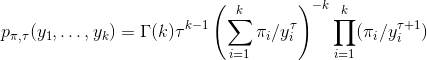Gumbel softmax pdf for temperature τ.

The main point of using Gumbel softmax is it makes everything fully differentiable. Let’s call 1-hot samples z and Gumbel soft samples y. Before when we sampled 1-hot vectors z we couldn’t back prop through dz/dπ since the sampling process is non-differentiable. With Gumbel softmax, we have reparameterized our sampling procedure such that dy/dπ is differentiable. For differentiable environments, this lets us backprop everything from the reward to the parameters of the model.

But wait! Why do we expect our environment to be differentiable? What do we do when it isn’t? Moreover, what if it doesn’t even make sense to input a soft sample y to our environment? For example if we’re dealing with an agent in gridworld, it has to go left, right, up or down, not a combination of the four directions! To deal with an environment that requires us to choose a single discrete action to act in the world, we make another approximation. So far we’ve only defined a way to build a k dimensional “soft” sample with all nonzero values. In order to act in the world, we construct a hard sample z as the 1-hot vector described by the argmax of y. For back propagation, we simply assume the derivative of z is equal tot he derivative of y.

In other words, we act in the world with the argmax of y but backprop using the entire (dense) vector. In general, this approximation is called “straight through.” On paper it might look a bit sketchy but empirically it works pretty well compared to REINFORCE methods.

The above diagram shows the main crux of Gumbel softmax. The action probabilities π are rewritten as α in the diagram. G is the sample from the Gumbel distribution. In a, we see that Gumbel max converts a single sampling process to sampling for all dimensions plus argmax. The sampling process has been reparameterized so that the actual sampling part (blue) is separate from the backpropagation path (backprop only goes through the α’s). Colloquially, Gumbel is responsible for “getting all the dimensions involved so we can send a training signal back through them.” In b, we see that the softmax part of Gumbel softmax is important because we don’t know how to differentiate through argmax operations but we do know how to differentiate through softmax.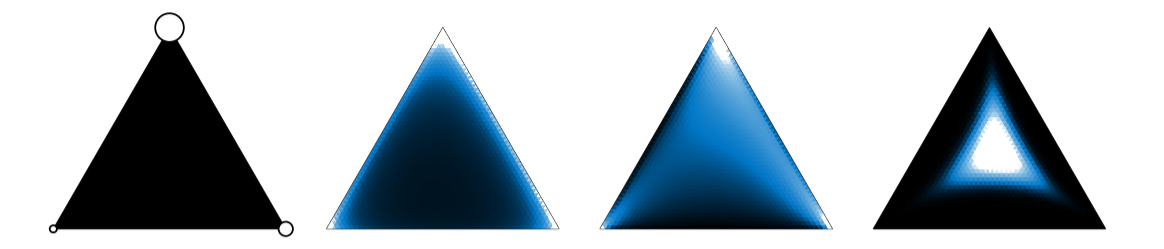Simplex in 3D. Visualization of Gumbel samples for a problem with 3 actions. The temperature is 0 for the left-most image and increases towards the right. For the 0 temperature case, samples must be one of the corners of the simplex (1-hot) because we are sampling directly from the multinoulli distribution.

Here is another interpretation of Gumbel softmax samples. These are diagrams of the Gumbel softmax probability density for k=3 dimensions. Recall that a simplex (subspace defined by the equation x_1 + x_2 + … = 1 where x are the values of each k dimensions) in N-dimensional space is a “triangle” lying in N-1 dimensions (here 2D). White means high probability density and black means low probability density. The pictures go from 0 temperature on the left to a high temperature on the right. The circles on the left-most picture show the relative probabilities of each class in the multinoulli distribution i.e. the values of vector π. We see that if we make the temperature higher, our samples look less like 1-hot vectors (further from the corners) and therefore don’t represent the multinoulli distribution as well.

I find that you can understand all the theory but won’t really know what’s going on unless you see an application in the wild. Consider applying the Gumbel softmax trick to a variational autoencoder (VAE). Given some input image, a VAE encodes it to a latent representation z and decodes it to a reconstructed image. Typically the latent variable is represented by a real-valued vector. Suppose we want the latent variable z of the VAE to be a 1-hot discrete vectors instead. This makes the model interpretable as each dimension of z could represent a particular class of objects. When a particular dimension of z is “on” (i.e. equals 1) we know that class is present in the image.

To train the discrete VAE, we apply the Gumbel softmax trick instead of the usual Reparameterization trick to differentiate through sampling z. Recall that the VAE loss is:

Where Q(z|x) is the posterior, P(z) is the prior and P(x|z) is the likelihood distribution. Let y be a soft sample drawn from the Gumbel softmax distribution parameterized by probabilities π. π are the probabilities of each dimension of z being on and is the output of the encoder. Recall that if z is a real-valued then the encoder would output mean and variance vectors instead. For discrete z, the encoder outputs the probabilities of each latent dimension being on. Using the Gumbel softmax trick, the loss is converted to the following equation:

We have replaced the real-valued latent vector with a soft sample y from a Gumbel softmax distribution. Otherwise the math looks the same. Schematically, the VAE now looks like:

The decoder now takes y as input instead of z. As usual backpropagation is done in two parts: from the discriminative loss D and the KL loss. After we have trained the discrete VAE, we can probe the decoder by passing in 1-hot vectors to find out what each dimension of the latent representation is.

### REBAR: Low-variance, unbiased gradient estimates for discrete latent variable models

In some sense REBAR is the successor of Gumbel softmax/Concrete. As we have discussed Gumbel softmax is a biased, low-variance (well at least lower than REINFORCE) gradient estimator. REBAR tries to one-up Gumbel softmax by being both unbiased and low-variance. The main disadvantage of REBAR is its implementation complexity. While Gumbel softmax can be implemented in a few lines of code in PyTorch, REBAR takes considerably more work. To understand REBAR, let’s first set up some notation and relate it back to what we already know about Gumbel softmax. REBAR is conceptually more complex than Gumbel softmax so for simplicity we will consider a Bernoulli variable instead of a multinoulli variable. Just as our multinoulli variable z was parameterized by a set of probabilites π, we will consider a Bernoulli variable b parameterized by a probability θ. The probability of b=1 is P(b|θ) and the probability of b=0 is one minus that.

Let’s begin by defining a list of relevant variables, this will be useful to refer back to during the discussion.

• b: a Bernoulli r.v. This is our discrete choice. b takes on the values {0, 1}. b is analogous to z in Gumbel softmax.
• z: the “logit” of the softmax, it is analogous to log(π+g) for our Gumbel softmax formulation. We’re using z here to mean something different than z in the Gumbel formulation. It is an simply intermediate value used to compute the sample, not the hard sample.
• y: the soft sample. We use y in a similar manner to our Gumbel softmax formulation. The logistic function plays the role of the softmax and is used to compute the soft sample from the logit.
• H(z): the Heaviside function, this function is 1 if z>0 and 0 if z<0. The heaviside function plays the role of the argmax for the Gumbel formulation. The hard sample is computed from the Heaviside function applied to the logit.
• u: a uniform random variable
• v: another uniform random variable

As before, our model will output a set of probabilities θ parameterizing the discrete random variable b. The goal is to sample b using θ, act in the environment with b, receive rewards, and learn to output better values of θ.

To derive the REBAR gradient estimator, we’re going to need to use a bunch of variables. I think it’s easiest if we start by defining the relationship between all of the them. Then during the REBAR derivation, we can refer back to these formulas.

Just as with Gumbel softmax, we can define logits based on the output of the neural network θ and a uniform random variable u.

The logit is key to computing a sample for our probability distribution. In particular, a “hard sample” can be computed by applying the Heaviside function to the logit.

A “soft sample” (the continuous relaxation) can be computed by applying the logistic function instead of the Heaviside function.

For the Gumbel softmax trick, this is the end of the story. Having defined a continuous relaxation of our sampling process, we can do backpropagation. To be concrete what this means, let’s define f as the environment function which determines the reward we receive. Our goal is to find the gradients of the reward with respect to the output of the model θ (once we’ve found this, we can propagate the gradients through the weights of the model).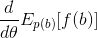Gradient of the unrelaxed model. This is the value we want to compute and REBAR will morph this equation into one with lower variance.

If f is differentiable, we can directly take the derivative:

The right hand side is the gradient estimator for Gumbel softmax. We replace the expectation over b with the expectation over u by noting that b = H(z(θ, u)). Furthermore, we make an approximation by using the soft sample y instead of b and σ_λ instead of H. Note that had we used the hard sample instead of the soft sample, the right hand side would be equal to the left hand side. We were able to bring the derivative into the expectation because we are now expecting over u which unlike b does not depend on θ. In this light, the Gumbel softmax gradient estimator can be seen as the Reparameterization trick applied to a continuous relaxation of the Bernoulli (multinoulli) distribution.

For the RELAX method, we need to do a bit more work. Let’s define another variable which is the after-the-fact logit. After-the-fact meaning given we already have a sample for b, we can infer the value of the logit that led to the sample b.

g is some function which we assume exists and maps the random variable b back to logits based on θ and a sample v from a uniform distribution. How we’ll use will be clear in a moment. Here is an overview of the dependency between the variables.

The only thing missing from this graph is the soft sample y. You can compute y from the logit z by replacing H with σ_λ.

To derive the REBAR gradient estimator, we need two tricks: the Reparameterization trick which we have already seen with the Gumbel softmax estimator and the REINFORCE trick. Recall that REINFORCE works by bringing the derivative inside the expectation and introducing the log p(b|θ) term. Also recall that we can introduce a baseline to the REINFORCE gradient estimator to reduce the variance without biasing it. A good baseline is one that is well correlated with the function f(b). The REINFORCE estimator with a baseline c:

This is the first step in deriving the REBAR gradient estimator. All that’s left to do is to determine a good baseline c. In other words, we want to construct something that looks like the second term on the right hand side. An idea is to use the gradient estimator of the relaxed model (where we have replaced b with y). After all, f(y) must be quite correlated with f(b) and should act as a good baseline. Here is the gradient of the relaxed model where we have replaced b with y = σ_λ(z):

Applying the REINFORCE trick gets us the right hand side. We want the right hand side to take the form of the baseline term in our REINFORCE estimator of the unrelaxed model. We have 2 problems.

1. The expectation is in terms of z and not b.
2. Even if we can make the expectation in terms of b, it’s not clear that setting c = f(σ_λ(z)) will leave the gradient unbiased.

First we tackle problem number 1; problem 2 will eventually sort itself out. It can be shown that by introducing the after-the-fact logit variable , we an rewrite equation * into a form that expects over b instead of z.

=g(v, b, θ) is a differentiable and deterministic function that maps b back to logit space. It can be shown that g exists for our current setup.

The second term takes the exact form we want for our baseline with c=E_p(v)[f(σ_λ())]. If we were to subtract our REINFORCE gradient for the unrelaxed model by the right hand side of equation **, we would have a baseline-subtracted REINFORCE estimator with an extra term.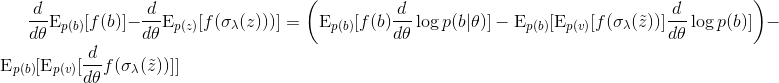Equation *** The right hand side is almost what we want: REINFORCE estimator with a baseline subtracted. However there’s an extra term so this gradient is biased.

This doesn’t work because remember REBAR is supposed to be an unbiased gradient estimator. To get around this problem, we invoke a common trick in mathematics: add and subtract the same thing! Consider:

This is equation *** with the relaxed gradient added back in. This equation is obviously unbiased because by adding and subtracting the same thing, we haven’t changed the original equation. The trick here is to replace the two relaxed gradient terms with a different reformulations. We replace the first two terms with equation ***. Next, we replace the third term with the Gumbel softmax approximation we derived earlier.

Recall that equation *** was derived by applying the REINFORCE trick to the relaxed gradient and equation **** was derived by applying the Reparameterization trick. In summary the REBAR gradient estimator is calculated by taking the REINFORCE estimator of the unrelaxed gradient, subtracting the REINFORCE estimator of the relaxed gradient, and adding back the Reparameterization estimator of the relaxed gradient. Phew!

Here is the REBAR gradient:

We’ve replaced expectations over b with expectations over u to get a formula that expects over u and v only (z is implictly dependent on u and is implicitly dependent on v). Finally b is calculated as H(z). The authors introduce a hyperparameter η which scales the baseline/control variate. I believe this is tuned. To relate REBAR back to Gumbel softmax, it’s interesting to note that the gradient estimator is unbiased for any temperature λ of the softmax function. The authors suggested learning λ during training rather than setting an annealing schedule ahead of time as you would for Gumbel softmax.

The algorithm for backpropation using the REBAR gradient is:

1. Sample u and v.
2. Compute z using u, θ. Compute b using z. Compute using b and v, θ.
3. Query the environment where ever there is a f() in the REBAR formula.
4. Backprop through the d/dθ in the REBAR formula. Note that when we do d/dθ f(σ_λ()) is a function of both θ and b. We should backprop through θ but not through b.

One limitation of REBAR is that it assumes you can evaluate the environment function f(b) at values of b between 0 and 1. This is apparent in the terms f(σ_λ()) and f(σ_λ(z)) which evaluates on the soft samples. This might not be possible in some settings like reinforcement learning.

### RELAX: Combining REBAR with a Q-Function Approximator

Just as REBAR is the successor to Gumbel softmax, RELAX is the successor to REBAR. Okay, this is not completely true since people have only compared the two methods for very specific problems and it’s not clear that one method is better than another in general. However RELAX is motivated by the shortcomings of REBAR. These shortcomings are: REBAR requires you to be able to evaluate the environment function f at intermediate points between discrete values, REBAR requires f to be differentiable, and REBAR requires multiple queries to f in a single gradient calculation. RELAX solves these problems at the cost of computing second order derivatives.

The authors introduce the term “surrogate function” to describe function used to baseline the gradient estimator. For REBAR, the surrogate function was f(σ_λ()). For RELAX, the surrogate function is learned. More specifically, the authors let the surrogate function be a second neural network c_ϕ parameterized by ϕ. Remember that a good baseline for the REINFORCE algorithm is one that is correlated with f(b). Therefore, we expect a good learned surrogate function c_ϕ to correlate with f(b). While RELAX is a generalization of REBAR to arbitrary surrogate functions, it might be conceptually easier to think of it as learning a Q-function c_ϕ alongside the policy network p_θ. However, as we will see there are some subtle differences between c_ϕ and the Q-function in reinforcement learning.

First let’s consider the case when b is a continuous random variable (this might seem a bit odd because we’ve been considering discrete b up to this point but RELAX has a novel use-case for continuous b as well as discrete b). Usually if b is continuous and f is differentiable, then we can use the Reparameterization trick. When f is non-differentiable, we are stuck with REINFORCE. The authors propose a gradient estimator called LAX for the continuous b, non-differentiable f case.

This is the same trick we applied for the REBAR algorithm where we added then subtracted the gradient of the surrogate function (replacing one term with the REINFORCE trick and the other term with the Reparameterization trick) to keep estimator unbiased. The only difference here is the surrogate is c_ϕ instead of f(σ_λ()). Because c_ϕ is just a neural network, it is differentiable and we can directly compute the last term in the equation. I have omitted the expectation over b. Notice that the LAX estimator does not require computing the derivative of f.

As with REBAR, LAX is unbiased. However, it’s actually not clear LAX will reduce the variance of your estimator. You reduce the variance when you subtract the REINFORCE baseline but it’s not clear that this will offset the additional variance from the surrogate Reparameterization term you’ve added. It’s encouraging to check that as c_ϕ approaches f, we get back the Reparameterization estimator (which usually has much lower variance than the REINFORCE estimator). Lastly, usually in RL you’re not allowed to use the Q-function as a baseline since it depends on the actions and will therefore bias the gradient. It’s common to use the value function as the baseline instead. LAX of course gets around this by adding a third term with the same expected gradient as the baseline back in. The comparison with RL will be made more clear in a bit.

Looking at the equation for LAX, we see that it only optimizes the parameters of the policy network. The question still remains as to how we want to optimize ϕ. Intuition tells us c_ϕ should be correlated with f and we can explicitly force c_ϕ towards f by minimizing the L2 loss [f(b)−c_ϕ(b)]². This is analogous to how the Q-function is trained in RL. However, recall that we have both a REINFORCE term and a Reparameterization term in LAX. Optimizing the baseline to lower the variance of the REINFORCE term runs the risk of increasing the variance of the Reparameterization term. Therefore let’s directly minimize the variance of the gradient, which is exactly the metric we want to reduce. In fact, we’ll see an example later where minimizing variance does better than having c_ϕ equal f.

Here ĝ is the gradient, or derivative respect to θ of the expected reward f(b). I apologize for the change in notation but θ now refers to the parameters of the policy network rather than the probability that b=1. Can LAX have lower variance than the Reparameterization trick? It seems like it can. We said that when c_ϕ approaches f, we’ll get back the Reparameterization trick. Then we said there’s a better way to optimize c_ϕ than forcing to be as close to f as possible. This suggests that a well optimized c_ϕ can lead to a gradient estimator with lower variance than the Reparameterization trick estimator.

Here is the LAX algorithm:

If b is Gaussian for example, then reparameterized sampler would use a normal random variable. We update the policy and surrogate networks simultaneously. Furthermore, to update the surrogate network we need to compute second order derivatives dg_θ/dϕ.

Now let’s frame LAX in the context of RL. For simplicity consider a game where each episode goes on forever. One of the most popular RL algorithms is advantage actor-critic (A2C) which is just a variant of REINFORCE:

Here the baseline can be interpreted as a learned value function c_ϕ(s_t). Now let’s consider LAX in the RL setting:

Here the baseline c_ϕ depends on the action and can be interpreted as a learned Q-function. The gradient is unbiased because we add the Reparameterization gradient of the surrogate function back in. All in all, we have an unbiased gradient estimator that benefits from a baseline which utilizes action information.

Finally let’s discuss the discrete-action case. The authors call this algorithm RELAX. Superficially RELAX is exactly like REBAR but with c_ϕ instead of f(σ_λ()):

We’ve dropped the expectations over uniform random variables u and v for clarity but we still have to take these samples. We do the same thing to compute the RELAX gradient as the REBAR gradient. The only difference here is the surrogate function is guaranteed to be differentiable. Furthermore, we need to update the surrogate neural network alongside the policy network using the formula we derived for the LAX algorithm.

Now let’s consider a toy problem that highlights the strengths of the RELAX algorithm. In fact, we’ll show that it is sub-optimal for c_ϕ to be equal to f in terms of reducing the variance of the gradient estimator. Consider a Bernoulli random variable b parameterized by θ and a toy reward function:

where t is a fixed target. We’ve gone back to θ denoting the probability b=1. Note that the solution to this problem is actually a deterministic policy (stochastic policies are only optimal in adversarial and imperfect information settings). For example if t<0.5, then the pay-off is maximized if we always pick b=1 since each time we pick b=1 the reward is higher than choosing b=0. Therefore the solution is θ=1. The problem becomes harder to solve as t approaches 0.5, i.e. b=0.4,0.45,0.49,0.499 because for any given trial the reward signal is almost the same between b=0 and b=1. The authors considered the difficult problem of b=0.499. Because the environment function is known, we can evaluate it at intermediate values like 0.5 and use REBAR. Plots of the surrogate function for different gradient estimators are shown below. u is the probability of choosing b=1. The blue and green lines show the fixed surrogate function of REINFORCE and REBAR respectively. The red line shows the learned function for RELAX.

REBAR makes the mistakes of evaluating f(y)=(σ_λ(z)−t)² directly for its surrogate. This expression is 0 at σ_λ(z)=0.5 and large at 0 or 1. This roughly tells the algorithm that “values of θ around 0.5 are bad and values around 0 or 1 are good.” But this is simply not true! Picking the decision with higher pay-off (b=1) half the time (θ=0.5) is definitely better than picking it none of the time (θ=0). Recall that we have two d/dθ f() terms in REBAR. These terms have an extrema at θ=0.5 and can get our model stuck at that value which is not what we want. RELAX learns a much better surrogate for this problem and the d/dθ c_ϕ term pushes the solution towards the correct solution θ=1. The surrogate function reflects the pay-off much better; it’s supposed to increase monotonically with θ.

The reason REBAR fails here is because f is meant to be used on discrete values of b=0, 1 and using it at intermediate value sends the wrong training signal. In these situations, it might be better to optimize the surrogate c_ϕ to directly lower the variance rather than follow f.

The authors show that RELAX is able to learn faster and with more stability than A2C on various toy RL tasks. RELAX’s main advantage seems to be data efficiency: you reach a good model with fewer episodes of simulation. However, the authors don’t report a comparison of the running times of A2C and RELAX so chances are RELAX is slower in wall-clock time versus A2C.

In this blogpost I’ve talked about three new algorithms for optimizing neural networks with discrete choices: Gumbel softmax, REBAR, and RELAX. Gumbel softmax provides a simple but biased alternative to REINFORCE. RELAX and REBAR claim to solve discrete problems with unbiased and low variance gradients, but have yet to gain much traction due to the complexity of implementation. So which gradient estimator should I use for my model: Gumbel softmax, REBAR or RELAX? From my own experience it’s not clear that having bias in your gradient estimator is a deal breaker. Often times there are other challenges from the RL domain such as reward accreditation that are much more important to get right than getting an unbiased gradients. In might turn out to be the case the advantages of REBAR and RELAX will not be worth the extra implementation difficulty. In conclusion the verdict is still out on whether gradient estimators need to be unbiased to work well and I encourage you to try each of the methods yourself to find out.